This calculation model is only valid for SRD Inline process density meter and viscometer.

Model Name Brix: Valid for SRD

# 1. What it does?

Determines the pure sucrose content in water from line density and temperature

# 2. Application

Soft drinks, fruit juices, tomato concentrates – Beverage Industry - Cutting Oil – Machining/Industrial.

# 3. Maths

Below finds the mathematical formula for Brix degree calculation.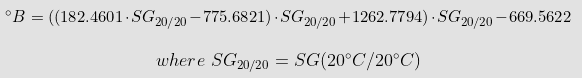To obtain the correct measurements for Brix degree, the SRD and SMET work together as a powerful tool for measuring density, which is an extremely significant variable. The user would need to input:

1. Values dataset of densities of different products at different temperatures
2. Input Water density at T2 (20°C – brix). Needed for Specific Gravity @20°C calculation. Compensated density is calculated with the values measured from the SMET.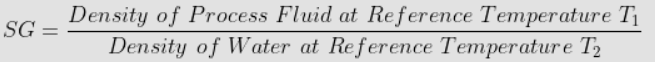3. Measure line density and line temperatures. Values are taken from SMET-SRD.
4. Convert the line density of process fluid to temperature compensated for density value at T1 (20°C –brix) – By using a dataset table from known products.

# 4. How to load models into the SMET?

The calculation tab allows configuring the SMET to run mathematical models for Viscosity, Density, and Concentration.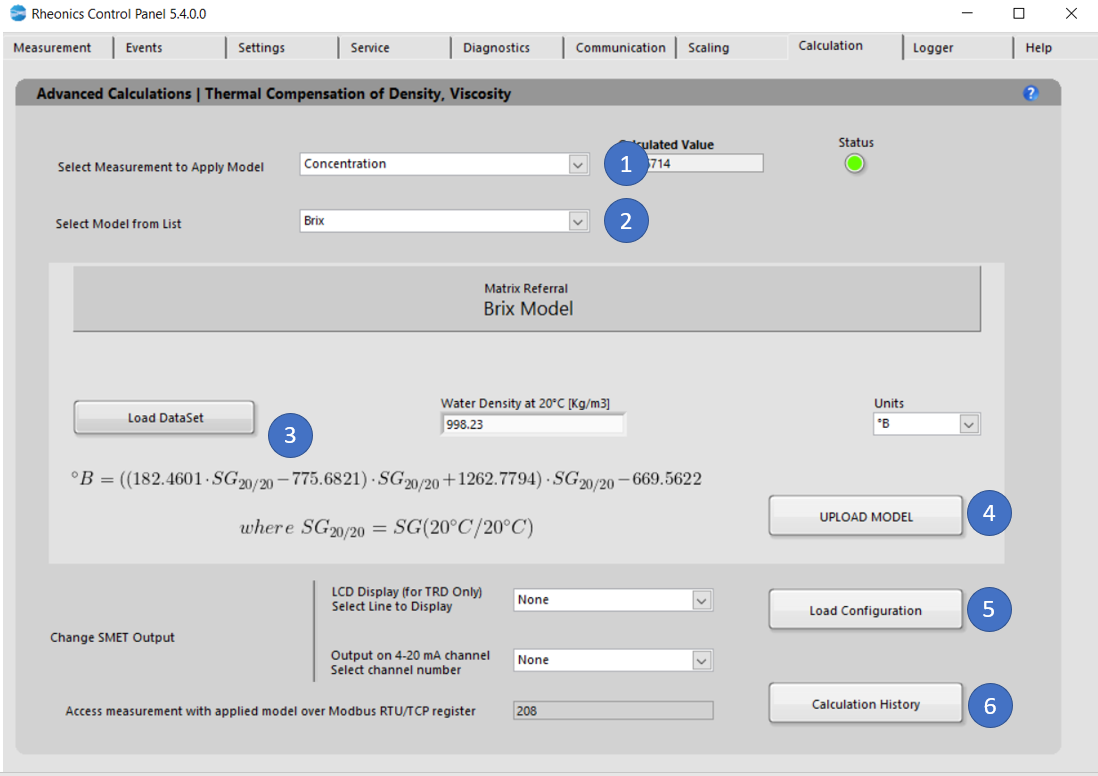Figure 1. Calculation tab for Brix degree model

1. In the Dropdown list “Select Measurement to Apply Model” select "Concentration" Model. That will modify the “Select Model from List” dropdown menu values.
2. In “Select Model from the list”, select the model you would like to use for the specific parameter. When you select the model, you will get more information about the specific equation used and the coefficients you will need to apply that model.  Select “Brix”
3. "Load Dataset" button. Using this operation is critical to obtaining the correct Brix degree measurement so that density and temperature as a result of the process are included in the table. The dataset must include at least the following instances:
• Five reference temperatures (at least)
• The density for each of four different product types at the five reference temperatures from the process (20 reference points in all, at least)
• The base temperature(20°C), must be one of the five reference temperatures.
• The inline density value is in-between the density values from the table otherwise output from SMET will be NaN.

# 5. Matrix Referral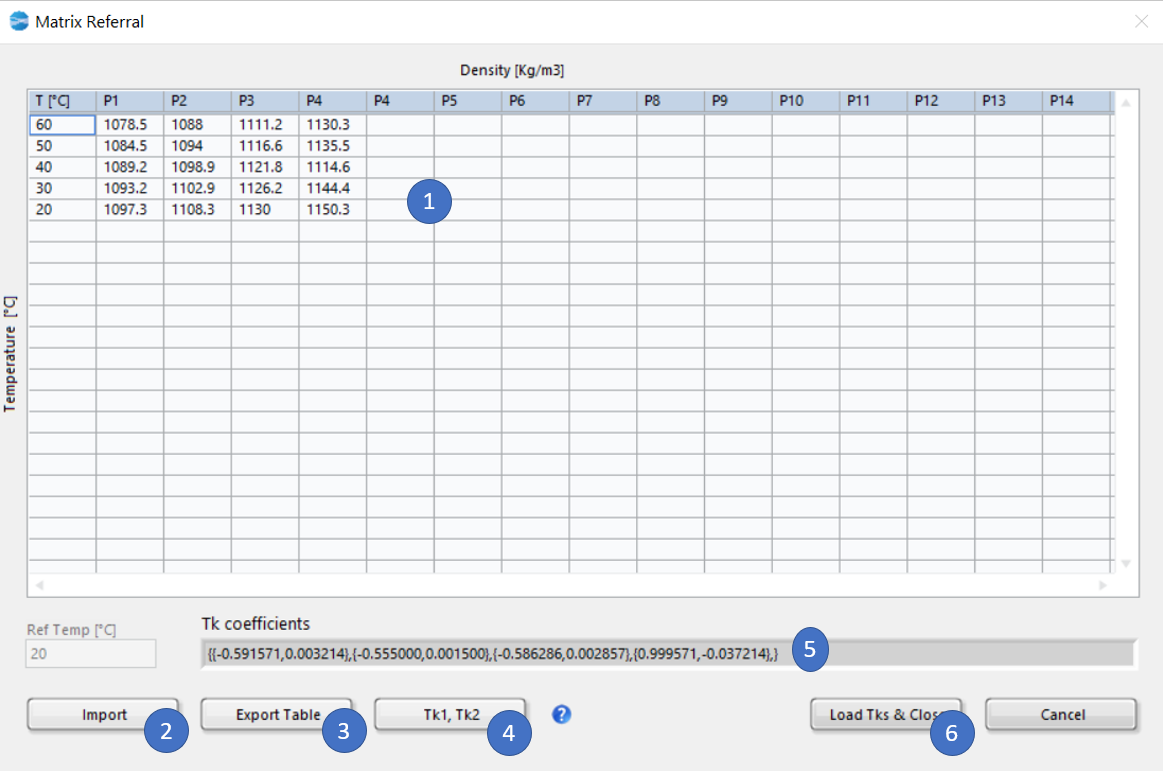Figure 2. Sample Dataset for Brix readings.

1. Dataset for beverage, the first column is for temperature. The rest of the columns should be each for a product "P", and the densities of that product at the different temperatures.
2. Import: Select dataset from an .csv sheet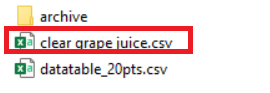Figure 3. Selecting dataset

3. Export: Export the current table to .csv

4. "Tk1, Tk2" button to create the coefficients that will be used on the model.

5. Calculated Tk coefficients by internal model.

6." Load Tks & Close" button to load these coefficients into the model

Click on "Upload Model" to load the model settings into SMET. If the operation was successful, the button should turn GREEN for a couple of seconds; otherwise, it should turn RED. This action will refresh the values for the Uncompensated and Compensated indicators. Below is a sample of Brix at the beginning of a fermentation process along with a typical fermentation curve demonstrating the full capabilities of the SRD monitoring system.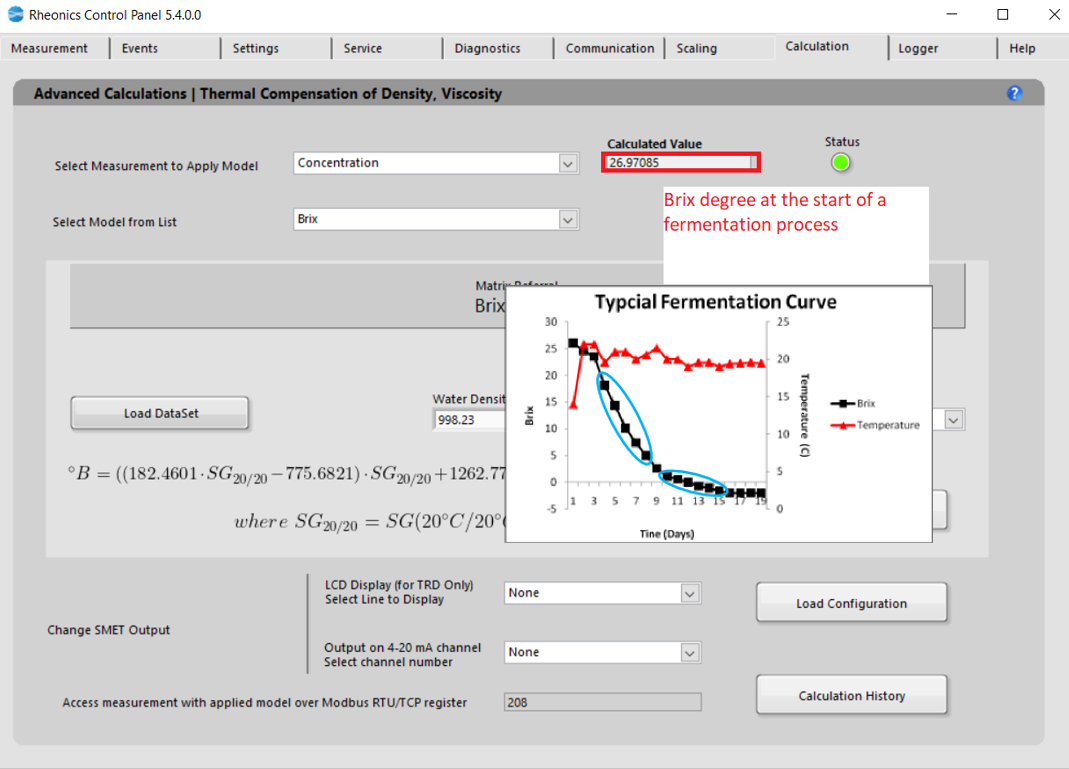Figure 4. Brix model loaded into the SMET.

6.1. Click “Load Configuration” to update the display and channel configurations in SMET.

• LCD Display

Select the line to display the parameter: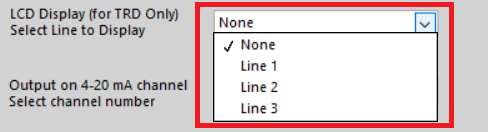Figure 5. Selecting read line for LCD display

Changes can be verified on the “Communication” Tab

• Analog outputs

Select analog channel to send data, this can be verified in “Service” Tab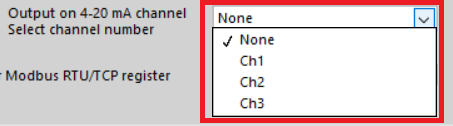Figure 6. Selecting analog output channel

• Modbus TCP/IP register

Measurement can always be accessed at the register address that is displayed.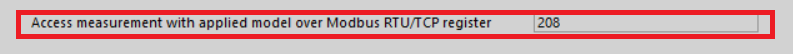• “Calculation History” button allows you to open a file where the last uploaded models and coefficients for these models are stored. This is for user reference.